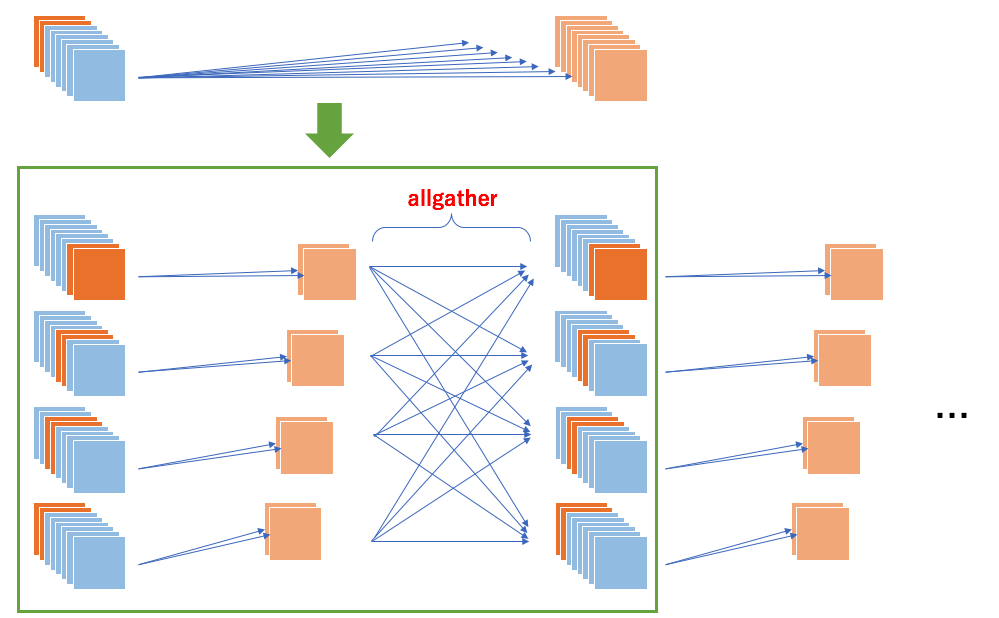# Example 3: Channel-wise Parallel Convolution¶

This is an example to parallelize CNN in channel-wise manner. This parallelization is useful with large batch size, or with high resolution images.The basic strategy is

1. to pick channels that each process is responsible for

2. to apply convolution, and

3. to use allgather to combine outputs of all channels into a single tensor

on each process. Parallel convolution model implementation could be like this:

class ParallelConvolution2D(chainer.links.Convolution2D):
def __init__(self, comm, in_channels, out_channels, *args, **kwargs):
self.comm = comm
self.in_channels = in_channels
self.out_channels = out_channels
super(ParallelConvolution2D, self).__init__(
self._in_channel_size, self._out_channel_size, *args, **kwargs)

def __call__(self, x):
x = x[:, self._channel_indices, :, :]
y = super(ParallelConvolution2D, self).__call__(x)
ys = chainermn.functions.allgather(self.comm, y)
return F.concat(ys, axis=1)

def _channel_size(self, n_channel):
# Return the size of the corresponding channels.
n_proc = self.comm.size
i_proc = self.comm.rank
return n_channel // n_proc + (1 if i_proc < n_channel % n_proc else 0)

@property
def _in_channel_size(self):
return self._channel_size(self.in_channels)

@property
def _out_channel_size(self):
return self._channel_size(self.out_channels)

@property
def _channel_indices(self):
# Return the indices of the corresponding channel.
indices = np.arange(self.in_channels)
indices = indices[indices % self.comm.size == 0] + self.comm.rank
return [i for i in indices if i < self.in_channels]


where comm is a ChainerMN communicator (see Step 1: Communicators).

ParallelConvolution2D can simply replace with the original Convolution2D. For the first convolution layer, all processes must input the same images to the model. MultiNodeIterator distributes the same batches to all processes every iteration:

if comm.rank != 0:
train = chainermn.datasets.create_empty_dataset(train)
test = chainermn.datasets.create_empty_dataset(test)

train_iter = chainermn.iterators.create_multi_node_iterator(
chainer.iterators.SerialIterator(train, args.batchsize), comm)
test_iter = chainermn.iterators.create_multi_node_iterator(
chainer.iterators.SerialIterator(test, args.batchsize,
repeat=False, shuffle=False),
comm)


An example code with a training script for VGG16 parallelization is available here.